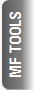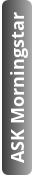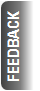# 5 ratios to measure risk and return

By Divyansh Awasthi |  14-10-14 |

A routine check on one's mutual funds is important. It helps to understand where one is and seems to be heading.  The primary method is to look at its returns vis-à-vis its benchmark index. But for a diligent investor, the analysis should not end there. There are several ratios that one should look at to further determine the scheme’s performance.

1)  Sharpe Ratio

Named after its founder William F. Sharpe, the Sharpe ratio helps to study the risk-adjusted performance of a mutual fund scheme. Technically, the ratio is defined as the excess returns of a scheme (over a risk-free rate) divided by the standard deviation of the scheme’s returns for a given period.

The risk-free rate can be 91 or 364-day Treasury-Bill issued by the government. The standard deviation is as a measure of risk; the higher the deviation, the higher the risk and hence, the lower the Sharpe Ratio. Simply put, this measure determines how the return of the scheme has compensated an investor for the risks it has taken. The higher the Ratio, the better compensation to the investor for bearing additional risk.

Let’s look at an illustration. Scheme Y returns 10% in a year while Scheme Z returns 8%. If the risk free rate is 4%, and the standard deviation of Y and Z is 8% and 4% respectively, then their respective Sharpe Ratios are 0.75 and 1. Thus, contrary to the initial inference that scheme Y was the superior performer (based on returns), scheme Z turns in a better performance on the risk-adjusted front.

But do note that this measure by itself provides little meaningful information. Using this measure makes sense when comparing schemes; the one with a higher Sharpe Ratio gives better returns for the same level of risk or the same returns with a lower level of risk. However, it is important to note that the schemes being compared should be of the same category. Comparing the Sharpe Ratio of a large-cap scheme with a sector scheme is fallacious as both funds are dissimilar.

As a rule of thumb, a ratio of 1 and above is good, 2 and above is very good and 3 and above is excellent

It is advisable to look at this ratio over several periods to assess how the scheme(s) have fared in different market cycles.

2)  Upside Capture and Downside Capture Ratio

These ratios, looked at in tandem, show you whether a fund has gained more or lost less than any benchmark and by how much.

The upside capture ratio, or UR, is calculated by taking the fund's monthly return for those time periods when its benchmark has posted positive returns and dividing the fund’s return by the benchmark’s return in the same month. Similarly, the downside capture ratio, or DR, is calculated by taking the fund's monthly return for negative return months for the benchmark and dividing it by the benchmark’s return.

A UR of above 100 implies that the fund has outperformed the benchmark during periods of positive benchmark returns while a DR of below 100 implies that the fund has lost less than its benchmark in negative benchmark return periods. Hence, a UR close to 100 and above and DR below 100 is desirable.

As investors, it is important to note that a just single ratio should not be used for making investment decisions. Any ratio should be analysed in conjunction with others before arriving at your optimal investment choice.

3) Standard Deviation

Standard Deviation, or SD, measures the dispersion of a set of data from its mean. The higher the SD, the more is the data away from the mean and vice versa. Simply put, this statistical measure represents the volatility or risk of an instrument. As the SD increases, so does the risk, which means that your investment has a chance to move either up or down in a much wider band than an instrument with a low SD. For instance, if an equity fund has given 15% returns on an average with an SD of 20 percentage points, its range of return can vary from -5% to 35%.

A high SD is not necessarily bad though, given the risk-taking capacity of an individual. Our readers will recall that SD is used in the denominator while calculating the Sharpe Ratio. So if your equity fund can consistently provide a high Sharpe Ratio for long periods even after having a high SD, it means that it is rewarding you well for the additional risk taken.

A related measure of deviation in performance of specifically index funds and Exchange Traded Funds (ETFs) is the tracking error (TE). This measure can single-handedly determine whether your index fund or ETF has done its job well or not. TE is the deviation between the returns of an instrument and the benchmark that it intends to replicate. Index funds and ETFs necessarily report a TE because of the expenses incurred in tracking their benchmark. But their efficiency in managing these expenses is shown by the TE; the lower the TE, the better.

4) Treynor Ratio

Named after Jack L. Treynor, this ratio is similar to the Sharpe Ratio in that it also measures the excess returns provided by an instrument over a risk free rate. But unlike Sharpe Ratio, which uses total risk (SD), Treynor Ratio uses market risk, represented by beta, in the denominator. Due to the use of beta in the calculation, this ratio is also known as reward-to-volatility ratio, since beta is the measurement of a security’s sensitivity to market movements (represented by a benchmark i.e. Sensex or Nifty for equities). As with the Sharpe Ratio, the higher the Treynor Ratio, the better.

5) Information Ratio

Information Ratio, or IR, is calculated by dividing the active return (returns of an instrument over a benchmark) by the volatility of those returns represented by TE. The IR can test the consistency of a fund manager as it determines whether a manager has beaten the benchmark by a large margin in a few months or by small margins every month. For a given level of risk taken, a higher active return will result in a higher IR, which in turn proves the consistency of a manager in delivering superior returns.

Apart from the ratios given above, another popular measure is the Sortino Ratio, which takes the difference between actual returns of a managed instrument and the required rate of return and divides it by downside deviation (SD of returns below the required or target return).

Jensen's Alpha, which determines the abnormal return of a fund over the theoretical expected return, is also popular.

But irrespective of the tool you use for evaluation, the bottom line remains the same, any health check makes use of several tools and tests, not just a few, and being a diligent investor, so should you.

Oct 15

Oct 15

Oct 15

Oct 14

Oct 13

## Recent from this Author

Oct 06

Sep 29

Sep 19

Sep 16

### Fundamental changes announced for ING MF schemes

Sep 12
Top